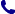• Contact
Choose interesting categories
I agree to receive promotional electronic communication(s).
Unsubscribe
• Institutional Clients
• Delivery
On-line access

# Bookstore

0.00 PLN
Bookshelf (0)

## Fractals

 Authors Publisher Productivity Press Inc Year 01/12/2019 Edition First Pages 194 Version hardback Readership level General/trade Language English ISBN 9781498741354 Categories Differential calculus & equations, Geometry, Analytic topology, Applied mathematics
\$192.35 (with VAT)
725.40 PLN / €162.96 / £148.42
Qty:
Delivery to United States

check shipping prices
Product to order
Delivery 5-6 weeks

## Book description

Most books on fractals focus on deterministic fractals as the impact of incorporating randomness and time is almost absent. Further, most review fractals without explaining what scaling and self-similarity means. This book introduces the idea of scaling, self-similarity, scale-invariance and their role in the dimensional analysis. For the first time, fractals emphasizing mostly on stochastic fractal, and multifractals which evolves with time instead of scale-free self-similarity, are discussed. Moreover, it looks at power laws and dynamic scaling laws in some detail and provides an overview of modern statistical tools for calculating fractal dimension and multifractal spectrum.

Fractals

Preface

Scaling, Scale-invariance and Self-similarity
Dimensions of physical quantity
Buckingham Pi-theorem
Examples to illustrate significance of P-theorem
Similarity
Self-similarity
Dynamic scaling
Scale-invariance: Homogeneous function
Power-law distribution

Fractals
Introduction
Euclidean geometry
Fractals
Space of Fractal
Construction of deterministic fractals

Stochastic Fractal
Introduction
A brief description of stochastic process
Dyadic Cantor set (DCS): Random fractal
Numerical Simulation
Stochastic fractal in aggregation with stochastic self-replication
Discussion and summary

Multifractality
Introduction
The Legendre transformation
Theory of multifractality
Multifractal formalism in fractal
Cut and paste model on Sierpinski carpet
Stochastic multifractal
Weighted planar stochastic lattice model
Algorithm of the weighted planar stochastic lattice (WPSL)
Geometric properties of WPSL
Geometric properties of WPSL
Multifractal formalism in kinetic square lattice

Fractal and Multifractal in Stochastic Time Series
Introduction
Concept of scaling law, monofractal and multifractal time series
Stationary and Non-stationary time series
Fluctuation analysis on monofractal stationary and non-stationary time series
Fluctuation analysis on stationary and non-stationary multifractal time series
Discussion

Application in Image Processing
Introduction
Generalized fractal dimensions
Image thresholding
Performance analysis
Medical image processing
Mid-sagittal plane detection

References

## We also recommend books801 777 223